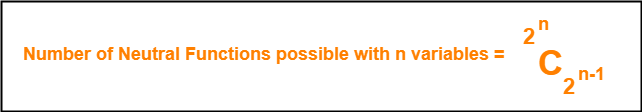# Neutral Functions | Minterms and Maxterms

## Minterms and Maxterms-

 For any function consisting of n Boolean variables, Number of minterms possible = Number of maxterms possible = 2n

## Minterms and Maxterms Examples-

The examples of minterms and maxterms are-

### Example-01:

For any function consisting of 2 Boolean variables A and B, we have-

• Number of minterms possible = 22 = 4.
• Number of maxterms possible = 22 = 4.

The following table shows the minterms and maxterms-

 A B Minterms Maxterms 0 0 A’B’ A + B 0 1 A’B A + B’ 1 0 AB’ A’ + B 1 1 AB A’ + B’

### Example-02:

For any function consisting of 3 Boolean variables A, B and C, we have-

• Number of minterms possible = 23 = 8.
• Number of maxterms possible = 23 = 8.

The following table shows the minterms and maxterms-

 A B C Minterms Maxterms 0 0 0 A’B’C’ A + B + C 0 0 1 A’B’C A + B + C’ 0 1 0 A’BC’ A + B’ + C 0 1 1 A’BC A + B’ + C’ 1 0 0 AB’C’ A’ + B + C 1 0 1 AB’C A’ + B + C’ 1 1 0 ABC’ A’ + B’ + C 1 1 1 ABC A’ + B’ + C’

## Neutral Functions-

 A neutral function is a function for which number of minterms and number of maxterms are same.

### Example-

The following function is an example of a neutral function-

f( A, B) = A ⊕ B

This is because this function has equal number of minterms and maxterms as shown by the following table-

 A B A ⊕ B 0 0 0 0 1 1 1 0 1 1 1 0

## Number Of Neutral Functions Possible-Here n = Number of Boolean variables in the function.

### Explanation-

• For n variables, total number of terms possible = number of combinations of n variables = 2n.
• Since maximum number of terms possible = 2n, so we choose half of the terms i.e 2n / 2 = 2n-1.
• We assign them the output logic ‘1’.
• We assign ‘0’ to rest half of the terms.
• Thus, number of neutral functions possible with n Boolean variables = C ( 2n , 2n-1 ).

## Problem-

Consider any function consisting of 2 Boolean variables A and B-

1. Calculate the total number of neutral functions that are possible.
2. Write all the neutral functions.

### Solution-

We know, number of neutral functions possible with n Boolean variables = C ( 2n , 2n-1 ).

Substituting n = 2, we get-

Number of neutral functions possible with 2 Boolean variables

= C ( 22 , 22-1 )

= C ( 4 , 2 )

= 6

Thus, total 6 Boolean functions are possible with 2 Boolean variables.

The 6 Boolean Functions are-

• f ( A , B ) = A
• f ( A , B ) = A’
• f ( A , B ) = B
• f ( A , B ) = B’
• f ( A , B ) = A ⊙ B
• f ( A , B ) = A ⊕ B

 Variables Boolean Functions A B A A’ B B’ A⊙B A⊕B 0 0 0 1 0 1 1 0 0 1 0 1 1 0 0 1 1 0 1 0 0 1 0 1 1 1 1 0 1 0 1 0

The above table clearly shows that for each function, number of minterms = number of maxterms.

So, they all are neutral functions.

To gain better understanding about Neutral Functions,

Watch this Video Lecture

Next Article- Self-Dual Functions

Get more notes and other study material of Digital Design.

Watch video lectures by visiting our YouTube channel LearnVidFun.

SummaryArticle Name
Neutral Functions | Minterms and Maxterms
Description
A neutral function is a function for which number of minterms and number of maxterms are same. Number of neutral functions possible with n Boolean variables = C ( 2n , 2n-1 ).
Author
Publisher Name
Gate Vidyalay
Publisher Logo